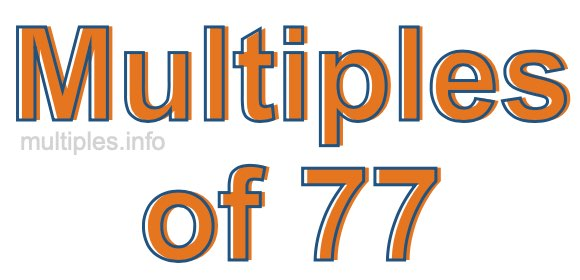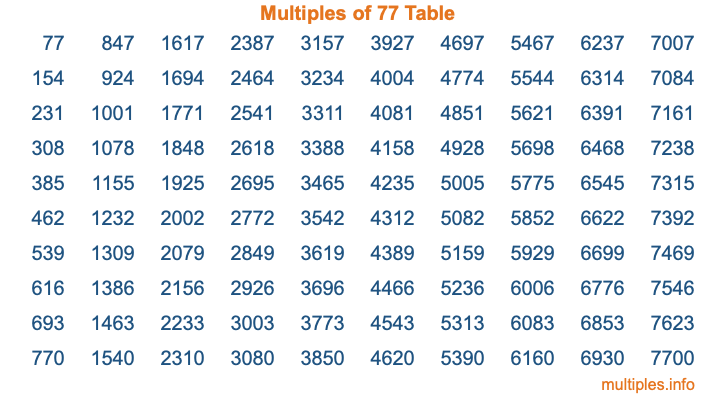Multiples of 77Welcome to the Multiples of 77 page. Here we will first teach you everything you will ever need to know about the multiples of 77, and then give you a study guide summary of everything we taught you to make sure you remember it all. Use this page to look up facts and learn information about the multiples of 77. This page will make you a multiples of seventy-seven expert!

Definition of Multiples of 77
Multiples of 77 are all the numbers that when divided by 77 equal an integer. Each of the multiples of 77 are called a multiple. A multiple of 77 is created by multiplying 77 by an integer.

Therefore, to create a list of multiples of 77, you start with 1 multiplied by 77, then 2 multiplied by 77, then 3 multiplied by 77, and so on for as long as you want. Thus, the list of the first five multiples of 77 is 77, 154, 231, 308, and 385. To see a larger list of multiples of 77, see the printable image of Multiples of 77 further down on this page. We also have a category where you can choose any nth multiple of 77.

Multiples of 77 Checker
The Multiples of 77 Checker below checks to see if any number of your choice is a multiple of 77. In other words, it checks to see if there is any number (integer) that when multiplied by 77 will equal your number. To do that, we divide your number by 77. If the the quotient is an integer, then your number is a multiple of 77.

Is  a multiple of 77?

Least Common Multiple of 77 and ...
A Least Common Multiple (LCM) is the lowest multiple that two or more numbers have in common. This is also called the smallest common multiple or lowest common multiple and is useful to know when you are adding our subtracting fractions. Enter one or more numbers below (77 is already entered) to find the LCM.

Check out our LCM Calculator if you need more details about the Least Common Multiple or if you need the LCM for different numbers for adding and subtraction fractions.

nth Multiple of 77
As we stated above, 77 is the first multiple of 77, 154 is the second multiple of 77, 231 is the third multiple of 77, and so on. Enter a number below to find the nth multiple of 77.

th multiple of 77

Multiples of 77 vs Factors of 77
77 is a multiple of 77 and a factor of 77, but that is where the similarities end. All postive multiples of 77 are 77 or greater than 77. All positive factors of 77 are 77 or less than 77.

Below is the beginning list of multiples of 77 and the factors of 77 so you can compare:

Multiples of 77: 77, 154, 231, 308, 385, etc.

Factors of 77: 1, 7, 11, 77

As you can see, the multiples of 77 are all the numbers that you can divide by 77 to get a whole number. The factors of 77, on the other hand, are all the whole numbers that you can multiply by another whole number to get 77.

It's also interesting to note that if a number (x) is a factor of 77, then 77 will also be a multiple of that number (x).

Multiples of 77 vs Divisors of 77
The divisors of 77 are all the integers that 77 can be divided by evenly. Below is a list of the divisors of 77.

Divisors of 77: 1, 7, 11, 77

The interesting thing to note here is that if you take any multiple of 77 and divide it by a divisor of 77, you will see that the quotient is an integer.

Multiples of 77 Table
Below is an image of the first 100 multiples of 77 in a table. The table is in chronological order, column by column. The first column has the first ten multiples of 77, the second column has the next ten multiples of 77, and so on.The Multiples of 77 Table is also referred to as the 77 Times Table or Times Table of 77. You are welcome to print out our table for your studies.

Negative Multiples of 77
Although not often discussed or needed in math, it is worth mentioning that you can make a list of negative multiples of 77 by multiplying 77 by -1, then by -2, then by -3, and so on, to get the following list of negative multiples of 77:

-77, -154, -231, -308, -385, etc.

Multiples of 77 Summary
Below is a summary of important Multiples of 77 facts that we have discussed on this page. To retain the knowledge on this page, we recommend that you read through the summary and explain to yourself or a study partner why they hold true.

There are an infinite number of multiples of 77.

A multiple of 77 divided by 77 will equal a whole number.

77 divided by a factor of 77 equals a divisor of 77.

The nth multiple of 77 is n times 77.

The largest factor of 77 is equal to the first positive multiple of 77.

77 is a multiple of every factor of 77.

77 is a multiple of 77.

A multiple of 77 divided by a divisor of 77 equals an integer.

77 divided by a divisor of 77 equals a factor of 77.

Any integer times 77 will equal a multiple of 77.

Multiples of a Number
Here you can get the multiples of another number, all with the same attention to detail as we did for multiples of 77 on this page.

Multiples of
Multiples of 78
Did you find our page about multiples of seventy-seven educational? Do you want more knowledge? Check out the multiples of the next number on our list!